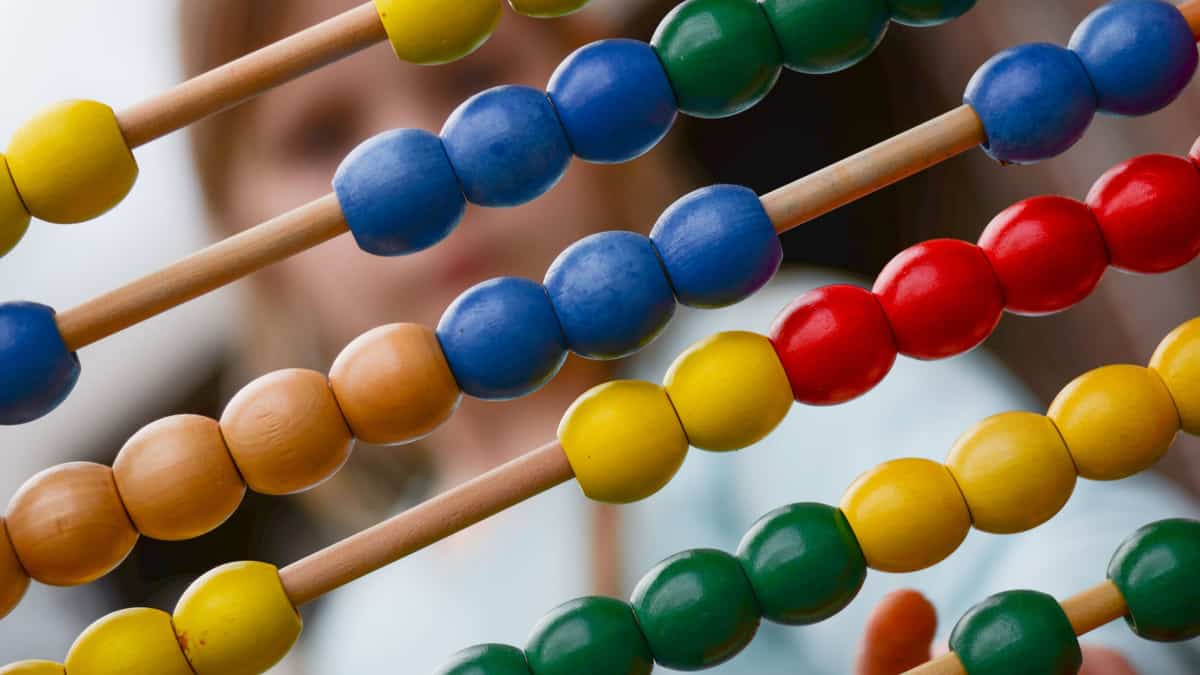# STEM Activities for Middle School Math

*We are reader-supported! This page may contain affiliate links. As an Amazon Associate we earn from qualifying purchases. The price to you remains the same.

Related post: Quick STEM Activities for 5th Grade Students

## For Young Students

Try games that have simple number recognition and counting at their core:

Cutting a big cookie or a big pizza into slices is the perfect way to teach your students about how fractions work. Be sure to talk about numerators and denominators to make sure all your students get the right amount of pizza!

Try out a Math Bingo game! Hearing numbers over and over again (and pairing them with correct squares on a bingo card) is the perfect way to reinforce the connection between words and symbols that we use in math problems. Math bingo is exciting and will get students engaged.

Have your students make paper clocks and practice telling time! This is a tricky one – students will benefit greatly from making their own clock and moving the hands around in a circle. This visual aid is critical to helping students understand how to tell time from a circle.

Guesstimation jar is the sweet way to get students engaged with probability. Put a number of small objects in a container and then having students guess as to how many objects are in the jar. This is a great way to teach about probability

As an added measure, weigh the ‘full’ jar each week to compare the density of objects. Because the jar will be the same size each week, the weight will vary only because of the density of the objects in the jar.

## For Older Students

In high school, try these set of activities:

Try measuring the time in between bounces of a ball and then graphing the results of your study! Students should look for a diminishing amount of time in between each bounce of the ball, and then graph out the parabola of each bounce. This is an easy way to model the acceleration of gravity against a “falling object.” Use a graphing calculator to help students quickly see the regression line that best fits the data gathered.

Study probability of numbers on dice to analyze the basics of probability. How does having more dice further randomize the outcomes of a roll? What is the highest number possible on a roll of dice?  These simple objects are fascinating to study how randomness is introduced into a game.

The first point that should be noted is that there are six possible configurations on the dice that yield the “7” combination. There is 6:1, 5:2, 4:3, 3:4, 2:5, and 1:6. This is important in analyzing probability in any game utilizing two dice. But first, we should study the number of combinations on the dice.

Study a classic game and discuss the hidden probabilities therein. The Monopoly board poses an interesting data set of probabilities that have been used for generations. The positions’ values have not changed much since the game’s inception decades ago.

This family-friendly, Atlantic City property trading game will be our playground for teaching a bit of math.

One important insight of this Monopoly board is that it is important to build three houses on the orange color group, which is close to the ‘jail’. People go to ‘jail’ frequently in this game. The worst investment is to own just one of the purple group, the lowest value property group – owning just one of these properties takes over 1,000 turns to return a profit.

Develop your own probability tables for Monopoly or another classic game. Have your students analyze the following questions:

• Which spaces cost the most in the game?
• Which spaces cost the least in the game?
• How quickly do you see a return (profit) on your investment for the Green monopoly?
• Which spaces are the “best” investments in the game?
• Which spaces are the “worst” investments in the game based on the return rate?

A data-driven approach to the game should understand that, if the combinations on the die are predictable, so too are the total odds of landing on a particular space given every position on the board.

• The goal of the exercise should be to develop a strategy for a game given the data available on the board.
• The first table is the breakeven table.
• This shows how many opponent rolls it will take before you make enough money back from people landing on your property.
• Compare this table against overall costs, and then plot out a strategy that changes as your money value changes during the game.

No matter what activity you are using to stimulate thought in your students, know that any activity you can use to teach math goes a long way to destigmatizing and engaging students. Anything is better than a timed skills test!